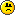Roman Forum
Would you like to react to this message? Create an account in a few clicks or log in to continue.##Table after Hemel Hempstead

Attendance 916

A good attendance but still not many here with points . . . . . . so a frustrating day on and off the pitchBenE    50 + 5 = 55
Bristol Mike   35 + 20 = 55
Ma Gibbons   55 + 0 = 55
Rabbit  20 +15 = 35
Ashley 30 + 0 = 30
Claire K  20 + 10 = 30
LB    25 + 0 = 25
Midsomer-Chris  25 + 0 = 25
Comrade Powell  15 + 0 = 15
Kermit   15 + 0 = 15
Luton Roman    15 + 0 = 15
Sean    15 + 0 = 15
Beau Nash   10 + 0 = 10
Midsomer-Steve    10 + 0 = 10
Azlimey 0 + 0 = 0
Maurice Ashman 0
PhilB1973   0 + 0 = 0
2Weirdtown   0 + 0 = 0Midsomer-chris

Posts : 1035
Join date : 2014-02-21
Age : 63Permissions in this forum:
You cannot reply to topics in this forum